Conventions

A "convention" is "just the way a thing is done!" Unfortunately, sometimes conventions in math are glossed over, and you're expected somehow "to just know" what they are. If you, like me, missed some of the conventions that relate the geometry and trigonometry, please review the following.

In geometrical pictures (or "figures", in the parlance), points are customarily labelled with capital Latin letters such as A, B, and C. Straight lines, and especially segments, are often labelled with lower-case Latin letters, such as a, b, and c, but straight lines are sometimes also labelled as subscripted ells, such as L1 for "line one".

Corners of figures, such as the corners of squares, are called "vertices" (VURR-tuh-seez); the singular is "vertex" (VURR-teks).

 If the meaning is clear, an angle may be referred to by just the point at its vertex, such as ∠C for the right angle show here: Properly, angles should be named completely, such as ∠BCA.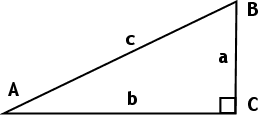If you're not sure that your meaning will otherwise be clear, or if you're not sure which naming convention your instructor prefers, use the three-letter method. That way, your meaning will always be clear.

In triangles, angles and opposite sides are usually corresponding upper- and lower-case Latin letters, as displayed in the picture above. The angle at A is opposite the side a, the angle at B is opposite the side b, and so forth. If your book does not provide specifications of orientation, such as a picture showing the labelled sides and angles or a worded description, you should probably assume this same-letter, different-case oppositional orientation.

To indicate the length of a line segment AB, you should use the absolute-value notation: |AB| = 3 cm. But many texts omit this, using AB to refer to both the segment and its length. To indicate the measure (the size) of an angle, you should use the m() notation: m(∠BCA) = 90°. But many texts omit this notation, too.

 Arrows on lines are used to indicate that those lines are parallel.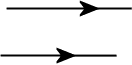If there is more than one pair of parallel lines, additional arrow-heads will be used. So this picture shows that p is parallel to q and r is parallel to s.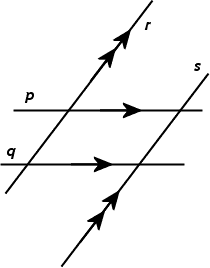Congruent angles (angles having the same measure or angle size) are indicated with arcs.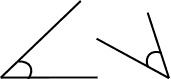If there is more than one pair of congruent angles, additional arcs will be used. So this picture shows that angle A is congruent to angle X and angle B is congruent to angle Y.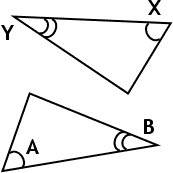Congruent segments (segments or polygon sides having the same length) are indicated by tick-marks. If there is more than one pair of congruent segments, additional tick-marks will be used. So this picture shows that side AB is congruent to side CD and side DA is congruent to side BC.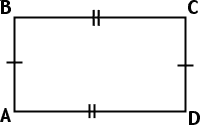Other notation, such as for rays, arcs, etc, is usually defined in the text. Unfortunately, as old as geometry is, the notation does not seem, even today, to be entirely standardized. So pay particular attention to how your book does things, so you can follow along, but don't be surprised if your instructor does something else.

For trigonometric functions, powers are indicated directly on the function names. For instance, "the square of the sine of beta" is written as sin2(β), and this notation means [sin(β)]2. Multipliers on the variable go inside the argument: sin(2β) does not mean the same thing as sin2(β). Some texts omit the function-notation parentheses, writing sin2β and sin2β, which can lead to confusion, especially when these expressions are hand-written. Try to remember to use the parentheses, so you can be clear in your own work. Also, try not to get in the lazy habit of omitting the arguments of the functions, writing things like sin2 + cos2 = 1, as this will lead to severe problems when the argument is not something simple like just "x".

The final "convention" I'll mention is really an assumption that you should remember not to make: Do not assume that pictures are "to scale"!

Original URL: https://www.purplemath.com/modules/conventn.htm